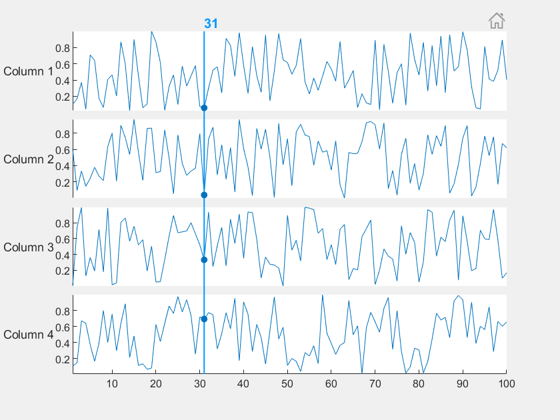# Greetings, Anyway to help to create ridgeline by MATLAB

45 views (last 30 days)
Yaser Khojah on 26 Nov 2019
Greetings,
Anyway to help to create ridgeline by MATLAB as the attache link please?
Adam Danz on 26 Nov 2019
Edited: Adam Danz on 26 Nov 2019
What release of matlab are you using? That's one of the options to fill in when asking a question and it's an important thing for us to know.

Adam Danz on 26 Nov 2019
Edited: Adam Danz on 28 Nov 2019
Here's a demo that creates a number of guassian distributions as input. The code produces an appropriate number of contiguous subplots where you can set the left, right, upper, and lower margins. Then it uses histfit() to compute and plot the density functions of each data. The code extracts the (x,y) values of the density curve and uses them to form a colored patch which replaces the histfit() plots. The axis limits and linked and some plot cosmetics are done to make the plot similar in appearance to the link you provided.
% Generate n distributions
n = 8; % number of distributions
mu = linspace(0,100,n);
sd = (rand(size(mu)) +1).*2;
nSamp = 100; %number of samples per dist.
data = arrayfun(@normrnd,mu,sd,ones(size(mu)),nSamp.*ones(size(mu)),'UniformOutput',false); % req. stats & ML toolbox
yLabs = num2cell(char(64+cumsum(ones(1,n))));
Now we have two key input variables.
1. data which is a 1 x n cell array where each element is a 1xm vector of data that will be used to compute a distribution.
2. yLabs : a 1 x n cell array of characters used to label each distribution along the y axis.
% Generate figure.
fh = figure();
% Compute axes positions with contigunous edges
n = numel(data);
margins = [.13 .13 .12 .15]; %left, right, bottom, top
height = (1-sum(margins(3:4)))/n; % height of each subplot
width = 1-sum(margins(1:2)); %width of each sp
vPos = linspace(margins(3),1-margins(4)-height,n); %vert pos of each sp
% Plot the histogram fits (normal density function)
% You can optionally specify the number of bins
% as well as the distribution to fit (not shown,
% see https://www.mathworks.com/help/stats/histfit.html)
% Note that histfit() does not allow the user to specify
% the axes (as of r2019b) which is why we need to create
% the axes within a loop.
% Otherwise we could use tiledlayout() (>=r2019b)
% https://www.mathworks.com/help/matlab/ref/tiledlayout.html
subHand = gobjects(1,n);
histHand = gobjects(2,n);
for i = 1:n
subHand(i) = axes('position',[margins(1),vPos(i),width,height]);
histHand(:,i) = histfit(data{i});
end
% Extend density curves to edges of xlim and fill.
% This is easier, more readable (and maybe faster) to do in a loop.
xl = xlim(subHand(end));
colors = jet(n); % Use any colormap you want
for i = 1:n
x = [xl(1),histHand(2,i).XData,xl([2,1])];
y = [0,histHand(2,i).YData,0,0];
fillHand = fill(subHand(i),x,y,colors(i,:),'FaceAlpha',0.4,'EdgeColor','k','LineWidth',1);
% Add vertical ref lines at xtick of bottom axis
arrayfun(@(t)xline(subHand(i),t),subHand(1).XTick); %req. >=r2018b
ylh = ylabel(subHand(i),yLabs{i});
set(ylh,'Rotation',0,'HorizontalAlignment','right','VerticalAlignment','middle')
end
% Cosmetics
% Delete histogram bars & original density curves
delete(histHand)
% remove axes (all but bottom) and
% add vertical ref lines at x ticks of bottom axis
set(subHand(1),'Box','off')
arrayfun(@(i)set(subHand(i).XAxis,'Visible','off'),2:n)
set(subHand,'YTick',[])
set(subHand,'XLim',xl)Jacqueline Chrabot on 19 Jul 2021
I'm trying to reuse this code, to make a plot, except my data is set up in a field where y is depth, x is time and the data fills in columns 1-12 of chlorophyll data. I'm trying to fit gaussian for each colomn and then display them all in the graph you showed. Can you help with this?

Image Analyst on 28 Nov 2019
Perhaps you'd be interested in stackedplot(): Pick of the Week>> doc stackedplot
Introduced in R2018b.

Santiago Benito on 21 Apr 2020
Edited: Santiago Benito on 21 Apr 2020
Hi there,
Maybe it's a little late, but I stumbled upon the same problem. I really wanted the plots to overlap, so I did the following:
% Number of data plots
n = 8;
% Sample points
N = 100;
% Distribution, example data
distName = 'normal';
mn = linspace(0,1,n);
% Allocate a matrix to store the dataset
yData = zeros(N,n);
% Plot options
mini = -0.3;
maxi = 1.3;
overlap = 0.4;
% Create the data
for ii = 1:n
distCell = makedist(distName,'mu',mn(ii),'sigma',0.1);
yData(:,ii) = pdf(distCell,linspace(mini,maxi,N));
end
% Get the position of each dataset
y = cumsum(max(yData,[],1))*(1-overlap);
% Create the figure with patch & plot
figure, hold on
for ii = n:-1:1
patch([linspace(mini,maxi,N),-mini],[yData(:,ii)+y(ii);y(ii)],mn(ii),...
'EdgeColor','none','FaceAlpha',0.8)
plot(linspace(mini,maxi,N),yData(:,ii)+y(ii),'k','LineWidth',1)
end
hold off
% Other stuff
colormap(spring)
yticks(y)
yticklabels({'A','B','C','D','E','F','G','H'})
xlim([mini,maxi])
The result:Some thoughts:
• This is not as elegant as the other solutions, but works for me.
Cheers!
Adam Danz on 23 Apr 2020
Edited: Adam Danz on 23 Apr 2020
The colorbar in the example above is a bit confusing. There are two yellows that have different values. The lower colorbar should be based on something like the winter colormap which doesn't have intersecting colors with the spring colormap. It's not clear why 2 colormaps/colorbars are needed.